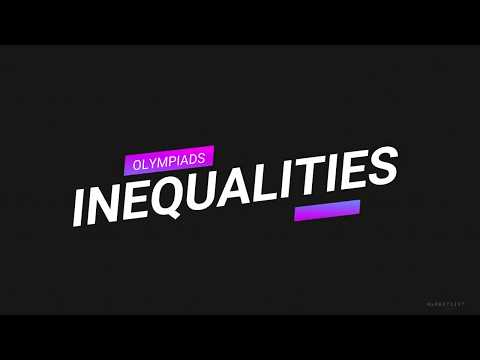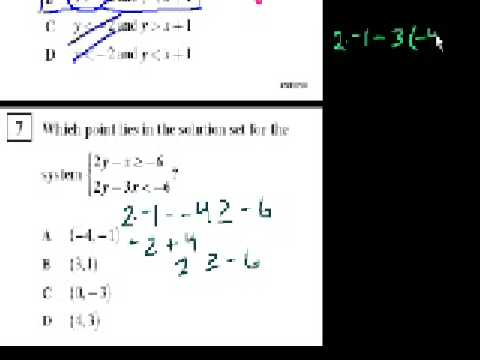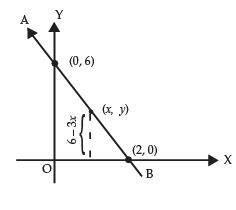Courses

# Test: Inequalities- 1

## 10 Questions MCQ Test IBPS Clerk Prelims - Study Material, Mock Tests | Test: Inequalities- 1

Description
This mock test of Test: Inequalities- 1 for Banking Exams helps you for every Banking Exams entrance exam. This contains 10 Multiple Choice Questions for Banking Exams Test: Inequalities- 1 (mcq) to study with solutions a complete question bank. The solved questions answers in this Test: Inequalities- 1 quiz give you a good mix of easy questions and tough questions. Banking Exams students definitely take this Test: Inequalities- 1 exercise for a better result in the exam. You can find other Test: Inequalities- 1 extra questions, long questions & short questions for Banking Exams on EduRev as well by searching above.
QUESTION: 1

### For which values of x does this equation stands true: 3x2 – 7x – 6 < 0

Solution:
• At x = 0, inequality is satisfied. Hence, options (b) and (c) are rejected.
• x = 3 gives LHS = RHS and x = -0.66 also does the same.
• Hence roots of the equation are 3 and -0.66. Thus, option (d) is correct
QUESTION: 2

### For which values of x does this equation stands true x2 – 14x – 15 > 0 ?

Solution:

At x = 0 inequality is not satisfied.
Thus x = –1 and x = 15 are the roots of the quadratic equation.

Thus, option (d) is correct.

QUESTION: 3

### For which values of x does this equation stands true |x2 - 4x| < 5 ?

Solution:

At x = 0 inequality is satisfied, option(b) is rejected. At x = 2, inequality is satisfied, option (c) is rejected.

At x = 5, LHS = RHS.
At x = –1, LHS = RHS.

Thus, option (a) is correct.

QUESTION: 4

For which values of x does this equation stands true |x – 6| > x2 – 5x + 9 ?

Solution:
• At x = 2, inequality is satisfied.
• At x = 0, inequality is not satisfied.
• At x = 1, inequality is not satisfied but LHS = RHS.
• At x = 3, inequality is not satisfied but LHS = RHS.

Thus, option (b) is correct.

QUESTION: 5

For which values of x does this equation stands true |x2 – 2x| < x ?

Solution:
• At x = 1 and x = 3
LHS = RHS.
• At x = 2 inequality is satisfied.
• At x = 0.1 inequality is not satisfied. At x = 2.9 inequality is satisfied.
• At x = 3.1 inequality is not satisfied.

Thus, option (a) is correct.

QUESTION: 6

For which values of x does this equation stands true x2 –7x + 12 < |x – 4| ?

Solution:
• At x = 0, inequality is not satisfied, option (a) is rejected.
• At x = 5, inequality is not satisfied, option (b) is rejected.
• At x = 2 inequality is not satisfied.
Option (d) is rejected.

Thus, Option (e) is correct.

QUESTION: 7

For which values of x does this equation stands true |x2 – 2x| < x ?

Solution:
• At x = 1 and x = 3
LHS = RHS.
• At x = 2 inequality is satisfied.
• At x = 0.1 inequality is not satisfied. At x = 2.9 inequality is satisfied.
• At x = 3.1 inequality is not satisfied. .
• At x = 3, inequality is not satisfied but LHS = RHS.

Thus, option (d) is correct.

QUESTION: 8

For which values of x does this equation stands true 2 – x – x2 ≥ 0 ?

Solution:

At x = 0, inequality is satisfied.
Thus, options, (c) and (d) are rejected.

At x = 1, inequality is satisfied
Hence, we choose option (b).

QUESTION: 9

For which values of x does this equation stands true x2 – |5x – 3| – x < 2 ?

Solution:

At x = 0, inequality is satisfied, option (a) rejected. At x = 10, inequality is not satisfied, option (c) rejected.
At x = –5, LHS = RHS.
Also at x = 5, inequality is satisfied and at x = 6, inequality is not satisfied. Thus, option (c) is correct.

QUESTION: 10

For which values of x does this equation stands true |x2  – 3x| + x – 2 < 0 ?

Solution:

The options need to be converted to approximate values before you judge the answer. At x = 0, inequality is satisfied.
Thus, option (b) and (d) are rejected.
Option (a) is correct.

Track your progress, build streaks, highlight & save important lessons and more!

### Similar Content### Related tests